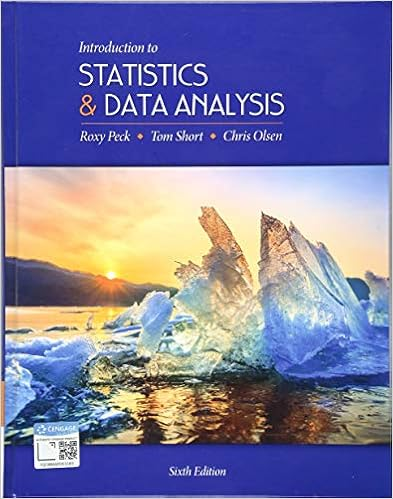• Test Prep
• 3

This preview shows page 1 - 2 out of 3 pages.

##### We have textbook solutions for you!
The document you are viewing contains questions related to this textbook.The document you are viewing contains questions related to this textbook.
Chapter 7 / Exercise 7.10
Introduction to Statistics and Data Analysis
Olsen/PeckExpert Verified
Your nameRecitation Section:(By signing your name here, youagree to abide by the GWU code of academic integrity.)STAT 1053.12 Fall 2017– Quiz 5 Version ATime: 20 minutesThursday, 10/12/171.(3 points)An urn contains 7 white, 5 blue and 8 red marbles. Someone picks 5 marbles atrandom without replacement from the urn.LetYbe the number of white marbles in thesample. DoesYfollow a binomial distribution? Explain.2.(2+4=6 points)It is known that 27% of the bottled-water plants fill their bottles with justtap water.An agency started sampling bottles until they found one that is filled with tapwater. LetXbe the number of bottles they sampled.a) DoesXfollow a binoial distribution? Why?b) Find the probability that at least 3 bottles need to be sampled.3.(3+4+4=11 points)In a multiple choice test there are 25 questions. Each problem has fiveoptions and only one of them is correct. A student is guessing all the answers as s/he did notstudy at all. LetYbe the number of questions s/he answered correctly.a) How isY
##### We have textbook solutions for you!
The document you are viewing contains questions related to this textbook.The document you are viewing contains questions related to this textbook.
Chapter 7 / Exercise 7.10
Introduction to Statistics and Data Analysis
Olsen/PeckExpert Verified
•••